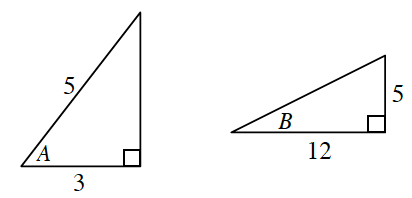### Home > PC3 > Chapter 12 > Lesson 12.2.1 > Problem12-99

12-99.

Given $\sec(A) = \frac { 5 } { 3 }$ and $\cot(B) = \frac { 12 } { 5 }$ where $0 < A$, $B < \frac { \pi } { 2 }$, determine the exact value of each of the following trigonometric expressions.

Draw a diagram of the two triangles. Use the Pythagorean Theorem to determine the length of the missing side.1. $\sin(A)$

1. $\cos(B)$

1. $\sin(A + B)$

Use the angle sum formula for sine:
$\sin(A + B) = \sin(A)cos(B) + \cos(A)\sin(B)$

$\text{sin}(A+B)=\frac{4}{5}\cdot \frac{12}{13}+\frac{3}{5}\cdot \frac{5}{13}=$

1. $\cos(A + B)$

Use the angle sum formula for cosine:
$\cos(A + B) = \cos(A)\cos(B) − \sin(A)\sin(B)$

1. $\tan(A + B)$

$\tan(A+B)=\frac{\sin(A+B)}{\cos(A+B)}$